## 换掉UUID，NanoID 更快、更短、更安全

|     |   0 浏览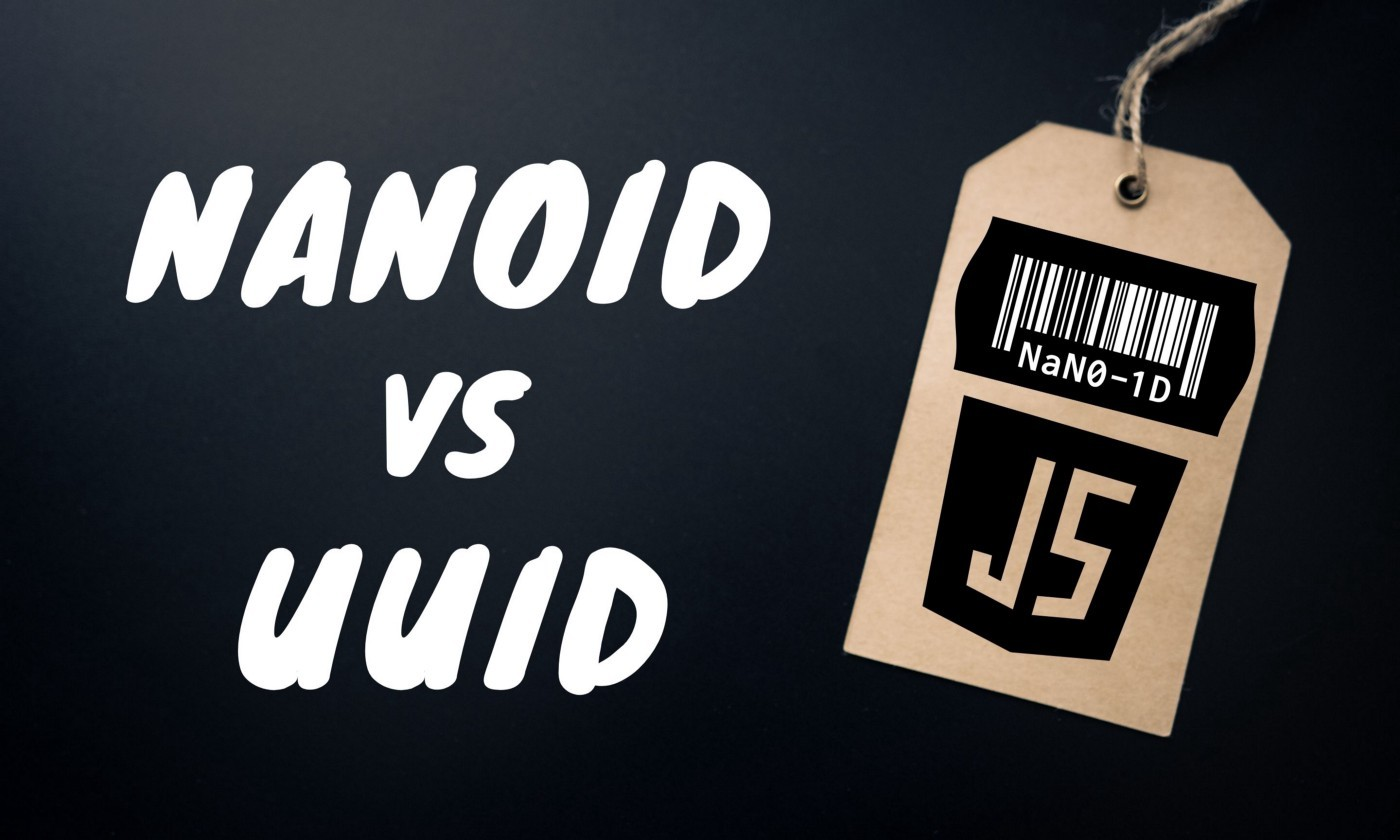UUID 是软件开发中最常用的通用标识符之一。然而，在过去的几年里，其他的竞品挑战了它的存在。

## NanoID的特点

### 3. 它既快速又紧凑

``````0123456789ABCDEFGHIJKLMNOPQRSTUVWXYZ_abcdefghijklmnopqrstuvwxyz-
``````

C#, C++, Clojure and ClojureScript, Crystal, Dart & Flutter, Deno, Go, Elixir, Haskell, Janet, Java, Nim, Perl, PHP, Python with dictionaries, Ruby , Rust, Swift

### 4. 兼容性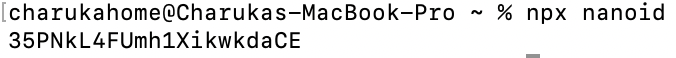``````import { nanoid } from ‘@reduxjs/toolkit’
console.log(nanoid()) //‘dgPXxUz_6fWIQBD8XmiSy’
``````

### 5. 自定义字母

NanoID 的另一个现有功能是它允许开发人员使用自定义字母表。我们可以更改文字或 id 的大小，如下所示：

``````import { customAlphabet } from 'nanoid';
const nanoid = customAlphabet('ABCDEF1234567890', 12);
model.id = nanoid();
``````

## 使用

### NodeJS

NanoID 每周的 NPM 下载量超过 1175.4 万，并且运行起来比 UUID 快 60%

• 安装

``````npm i nanoid
``````
• 使用

``````import { nanoid } from 'nanoid';
model.id = nanoid();
``````

### Java

• 添加依赖（方式一）

``````<dependency>
<groupId>com.aventrix.jnanoid</groupId>
<artifactId>jnanoid</artifactId>
<version>2.0.0</version>
</dependency>
``````
• 自定义工具类（方式二）；二选一即可

``````import java.security.SecureRandom;
import java.util.Random;

public final class NanoIdUtils {
public static final SecureRandom DEFAULT_NUMBER_GENERATOR = new SecureRandom();
public static final char[] DEFAULT_ALPHABET = "_-0123456789abcdefghijklmnopqrstuvwxyzABCDEFGHIJKLMNOPQRSTUVWXYZ".toCharArray();
public static final int DEFAULT_SIZE = 21;

private NanoIdUtils() {
//Do Nothing
}

public static String randomNanoId() {
return randomNanoId(DEFAULT_NUMBER_GENERATOR, DEFAULT_ALPHABET, 21);
}

public static String randomNanoId(Random random, char[] alphabet, int size) {
if (random == null) {
throw new IllegalArgumentException("random cannot be null.");
} else if (alphabet == null) {
throw new IllegalArgumentException("alphabet cannot be null.");
} else if (alphabet.length != 0 && alphabet.length < 256) {
if (size <= 0) {
throw new IllegalArgumentException("size must be greater than zero.");
} else {
int mask = (2 << (int) Math.floor(Math.log((double) (alphabet.length - 1)) / Math.log(2.0D))) - 1;
int step = (int) Math.ceil(1.6D * (double) mask * (double) size / (double) alphabet.length);
StringBuilder idBuilder = new StringBuilder();

while (true) {
byte[] bytes = new byte[step];
random.nextBytes(bytes);

for (int i = 0; i < step; ++i) {
int alphabetIndex = bytes[i] & mask;
if (alphabetIndex < alphabet.length) {
idBuilder.append(alphabet[alphabetIndex]);
if (idBuilder.length() == size) {
return idBuilder.toString();
}
}
}
}
}
} else {
throw new IllegalArgumentException("alphabet must contain between 1 and 255 symbols.");
}
}
}
``````
• 使用

``````String nanoId = NanoIdUtils.randomNanoId();
// aqiPmNG5kG6wtNIZP1g8d
``````

### 更多其他的语言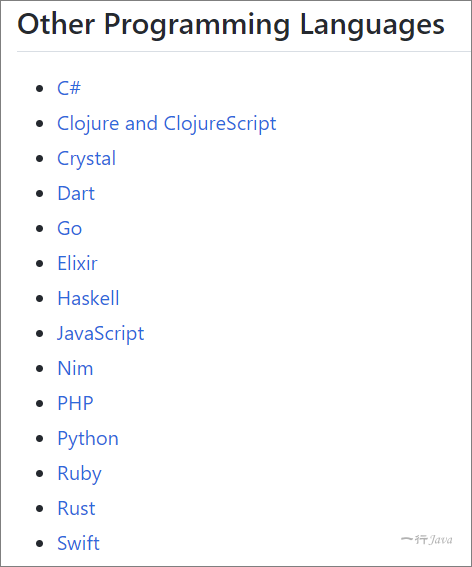## 未来趋势

NanoID 比 UUID 年轻了将近 7 年，而且它的 GitHub 星数已经比 UUID 多；下图显示了这两个之间的 npm 趋势比较，我们可以看到 NanoID 的上升趋势与 UUID 的平坦进展有强烈的对比。

https://www.npmtrends.com/nanoid-vs-uuid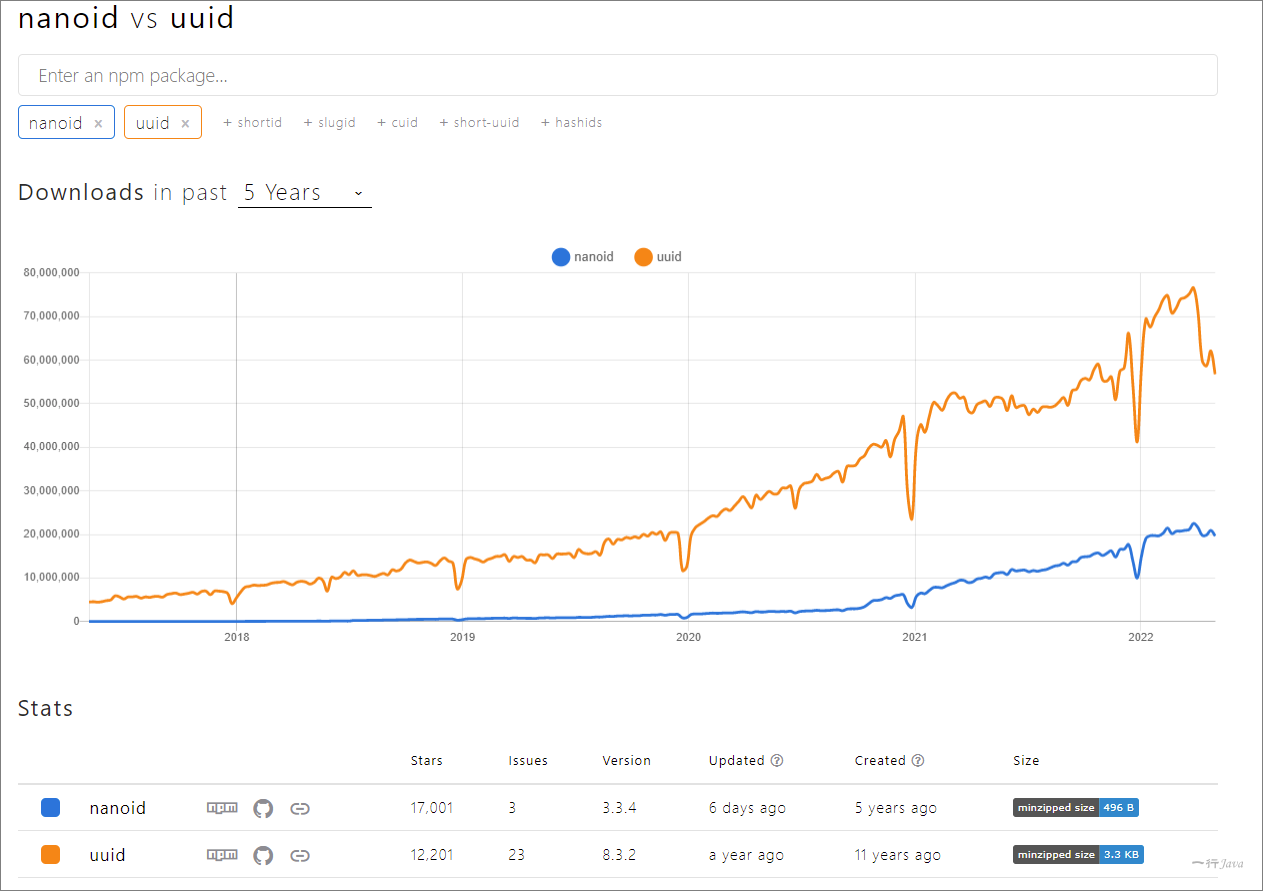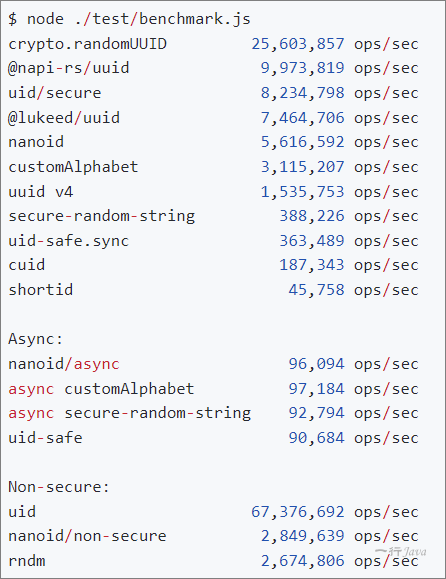http://dw-z.ink/1pgUC

https://www.npmjs.com/package/nanoid

https://github.com/aventrix/jnanoid